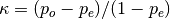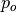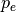Shortcuts

# Cohen Kappa¶

## Module Interface¶

### CohenKappa¶

class torchmetrics.CohenKappa(task: Literal['binary', 'multiclass'], threshold: float = 0.5, num_classes: = None, weights: Optional[Literal['linear', 'quadratic', 'none']] = None, ignore_index: = None, validate_args: bool = True, **kwargs: Any)[source]

Calculates Cohen’s kappa score that measures inter-annotator agreement. It is defined as.whereis the empirical probability of agreement andis the expected agreement when both annotators assign labels randomly. Note thatis estimated using a per-annotator empirical prior over the class labels.

This function is a simple wrapper to get the task specific versions of this metric, which is done by setting the task argument to either 'binary' or 'multiclass'. See the documentation of BinaryCohenKappa and MulticlassCohenKappa for the specific details of each argument influence and examples.

Legacy Example:
>>> target = torch.tensor([1, 1, 0, 0])
>>> preds = torch.tensor([0, 1, 0, 0])
>>> cohenkappa(preds, target)
tensor(0.5000)


### BinaryCohenKappa¶

class torchmetrics.classification.BinaryCohenKappa(threshold=0.5, ignore_index=None, weights=None, validate_args=True, **kwargs)[source]

Calculates Cohen’s kappa score that measures inter-annotator agreement for binary tasks. It is defined as.whereis the empirical probability of agreement andis the expected agreement when both annotators assign labels randomly. Note thatis estimated using a per-annotator empirical prior over the class labels.

As input to forward and update the metric accepts the following input:

• preds (Tensor): A int or float tensor of shape (N, ...). If preds is a floating point tensor with values outside [0,1] range we consider the input to be logits and will auto apply sigmoid per element. Addtionally, we convert to int tensor with thresholding using the value in threshold.

• target (Tensor): An int tensor of shape (N, ...).

Note

Additional dimension ... will be flattened into the batch dimension.

As output to forward and compute the metric returns the following output:

Parameters
Example (preds is int tensor):
>>> from torchmetrics.classification import BinaryCohenKappa
>>> target = torch.tensor([1, 1, 0, 0])
>>> preds = torch.tensor([0, 1, 0, 0])
>>> metric = BinaryCohenKappa()
>>> metric(preds, target)
tensor(0.5000)

Example (preds is float tensor):
>>> from torchmetrics.classification import BinaryCohenKappa
>>> target = torch.tensor([1, 1, 0, 0])
>>> preds = torch.tensor([0.35, 0.85, 0.48, 0.01])
>>> metric = BinaryCohenKappa()
>>> metric(preds, target)
tensor(0.5000)


Initializes internal Module state, shared by both nn.Module and ScriptModule.

### MulticlassCohenKappa¶

class torchmetrics.classification.MulticlassCohenKappa(num_classes, ignore_index=None, weights=None, validate_args=True, **kwargs)[source]

Calculates Cohen’s kappa score that measures inter-annotator agreement for multiclass tasks. It is defined as.whereis the empirical probability of agreement andis the expected agreement when both annotators assign labels randomly. Note thatis estimated using a per-annotator empirical prior over the class labels.

As input to forward and update the metric accepts the following input:

• preds (Tensor): Either an int tensor of shape (N, ...) or float tensor of shape (N, C, ..). If preds is a floating point we apply torch.argmax along the C dimension to automatically convert probabilities/logits into an int tensor.

• target (Tensor): An int tensor of shape (N, ...).

Note

Additional dimension ... will be flattened into the batch dimension.

As output to forward and compute the metric returns the following output:

Parameters
Example (pred is integer tensor):
>>> from torchmetrics.classification import MulticlassCohenKappa
>>> target = torch.tensor([2, 1, 0, 0])
>>> preds = torch.tensor([2, 1, 0, 1])
>>> metric = MulticlassCohenKappa(num_classes=3)
>>> metric(preds, target)
tensor(0.6364)

Example (pred is float tensor):
>>> from torchmetrics.classification import MulticlassCohenKappa
>>> target = torch.tensor([2, 1, 0, 0])
>>> preds = torch.tensor([
...   [0.16, 0.26, 0.58],
...   [0.22, 0.61, 0.17],
...   [0.71, 0.09, 0.20],
...   [0.05, 0.82, 0.13],
... ])
>>> metric = MulticlassCohenKappa(num_classes=3)
>>> metric(preds, target)
tensor(0.6364)


Initializes internal Module state, shared by both nn.Module and ScriptModule.

## Functional Interface¶

### cohen_kappa¶

torchmetrics.functional.cohen_kappa(preds, target, task, threshold=0.5, num_classes=None, weights=None, ignore_index=None, validate_args=True)[source]

Calculates Cohen’s kappa score that measures inter-annotator agreement. It is defined as.whereis the empirical probability of agreement andis the expected agreement when both annotators assign labels randomly. Note thatis estimated using a per-annotator empirical prior over the class labels.

This function is a simple wrapper to get the task specific versions of this metric, which is done by setting the task argument to either 'binary' or 'multiclass'. See the documentation of binary_cohen_kappa() and multiclass_cohen_kappa() for the specific details of each argument influence and examples.

Legacy Example:
>>> target = torch.tensor([1, 1, 0, 0])
>>> preds = torch.tensor([0, 1, 0, 0])
tensor(0.5000)

Return type

Tensor

### binary_cohen_kappa¶

torchmetrics.functional.classification.binary_cohen_kappa(preds, target, threshold=0.5, weights=None, ignore_index=None, validate_args=True)[source]

Calculates Cohen’s kappa score that measures inter-annotator agreement for binary tasks. It is defined as.whereis the empirical probability of agreement andis the expected agreement when both annotators assign labels randomly. Note thatis estimated using a per-annotator empirical prior over the class labels.

Accepts the following input tensors:

• preds (int or float tensor): (N, ...). If preds is a floating point tensor with values outside [0,1] range we consider the input to be logits and will auto apply sigmoid per element. Addtionally, we convert to int tensor with thresholding using the value in threshold.

• target (int tensor): (N, ...)

Additional dimension ... will be flattened into the batch dimension.

Parameters
Example (preds is int tensor):
>>> from torchmetrics.functional.classification import binary_cohen_kappa
>>> target = torch.tensor([1, 1, 0, 0])
>>> preds = torch.tensor([0, 1, 0, 0])
>>> binary_cohen_kappa(preds, target)
tensor(0.5000)

Example (preds is float tensor):
>>> from torchmetrics.functional.classification import binary_cohen_kappa
>>> target = torch.tensor([1, 1, 0, 0])
>>> preds = torch.tensor([0.35, 0.85, 0.48, 0.01])
>>> binary_cohen_kappa(preds, target)
tensor(0.5000)

Return type

Tensor

### multiclass_cohen_kappa¶

torchmetrics.functional.classification.multiclass_cohen_kappa(preds, target, num_classes, weights=None, ignore_index=None, validate_args=True)[source]

Calculates Cohen’s kappa score that measures inter-annotator agreement for multiclass tasks. It is defined as.whereis the empirical probability of agreement andis the expected agreement when both annotators assign labels randomly. Note thatis estimated using a per-annotator empirical prior over the class labels.

Accepts the following input tensors:

• preds: (N, ...) (int tensor) or (N, C, ..) (float tensor). If preds is a floating point we apply torch.argmax along the C dimension to automatically convert probabilities/logits into an int tensor.

• target (int tensor): (N, ...)

Additional dimension ... will be flattened into the batch dimension.

Parameters
Example (pred is integer tensor):
>>> from torchmetrics.functional.classification import multiclass_cohen_kappa
>>> target = torch.tensor([2, 1, 0, 0])
>>> preds = torch.tensor([2, 1, 0, 1])
>>> multiclass_cohen_kappa(preds, target, num_classes=3)
tensor(0.6364)

Example (pred is float tensor):
>>> from torchmetrics.functional.classification import multiclass_cohen_kappa
>>> target = torch.tensor([2, 1, 0, 0])
>>> preds = torch.tensor([
...   [0.16, 0.26, 0.58],
...   [0.22, 0.61, 0.17],
...   [0.71, 0.09, 0.20],
...   [0.05, 0.82, 0.13],
... ])
>>> multiclass_cohen_kappa(preds, target, num_classes=3)
tensor(0.6364)

Return type

Tensor

© Copyright Copyright (c) 2020-2023, Lightning-AI et al... Revision 6853c553`.

Built with Sphinx using a theme provided by Read the Docs.
Versions
latest
stable
v0.11.2
v0.11.1
v0.11.0
v0.10.3
v0.10.2
v0.10.1
v0.10.0
v0.9.3
v0.9.2
v0.9.1
v0.9.0
v0.8.2
v0.8.1
v0.8.0
v0.7.3
v0.7.2
v0.7.1
v0.7.0
v0.6.2
v0.6.1
v0.6.0
v0.5.1
v0.5.0
v0.4.1
v0.4.0
v0.3.2
v0.3.1
v0.3.0
v0.2.0
v0.1.0